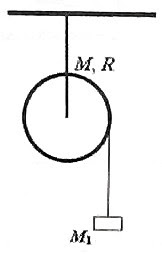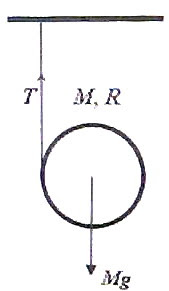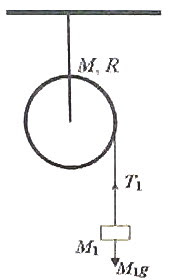## Pages

`“Life is like riding a bicycle.  To keep your balance you must keep moving.”–Albert Einstein`

## Sunday, October 4, 2009

### Answer to AP Physics C Free Response Practice Question on Rotation

In the post dated 30th September 2009 A free response question (for practice) on rotational motion for AP Physics C aspirants was given. As promised I give below a model answer for the same. The question also is given:A solid cylinder of mass M and radius R has a light inextensible string wound round it. The free end of the string is tied to a rigid support (Fig.) and the cylinder is released from its state of rest.

(a) Determine the acceleration ‘a of the cylinder as it moves down, unwinding the string.
The cylinder itself is now suspended from the support so that it is free to rotate about its axle. The friction at the axle of the cylinder is negligible. A mass M1 is now attached to the free end of the string wound round the cylinder as shown in the adjoining figure and the system is released from rest at time t = 0. Now answer the following questions (b), (c) and (d):(b) Determine the acceleration a1 of the mass M1 in terms of the given parameters.

(c) In terms of the given parameters obtain the angular acceleration of the cylinder when the mass M1 moves down, unwinding the string.

(d) Determine the kinetic energy of the cylinder at time t.(a) The weight Mg of the cylinder tries to drive it downwards while the tension T in the sring tries to oppose the downward motion (fig.). The net force on the cylinder is MgT. If the downward acceleration is a we have

MgT = Ma from which T = M (ga)

The torque produced by the tension T rotates the cylinder and produces an angular acceleration α (let us say) so that we have

TR = Iα where I is the moment of inertia (MR2/2) of the cylinder about its axis (axle). Therefore, TR = (MR2/2)α

But α = a/R so that TR = (MR2/2)(a/R)

Since T = M (ga), the above equation becomes

M (ga)R = (MR2/2)(a/R) from which (ga) = a/2 so that a = 2g/3(b) The force driving the mass M1 downwards is its weight M1g. The tension T1 in the sring tries to oppose the downward motion (fig.). The downward acceleration a1 of the mass M1 is therefore given by M1gT1 = M1a1 from which

T1 = M1(g – a1)

Since the torque produced by the tension T1 is T1R and this is responsible for the rotation of the cylinder, we have

T1R = Iα1 where α1 is the angular acceleration of the cylinder.

Substituting for T1 and the moment of inertia I, we have

M1(g – a1)R = (MR2/2)α1

Substituting for α1 = a1/R, the above equation becomes

M1(g – a1)R = (MR2/2)(a1/R)

Or, 2M1(g – a1) = Ma1, from which a1 = 2M1g/(2M1+M)

(c) The angular acceleration α1 of the cylinder when the mass M1 moves down, unwinding the string is given by

α1 = a1/R = 2M1g/ [(2M1+M)R]

(d) The kinetic energy of the cylinder at time t is due to its rotational motion and is equal to ½ Iω2 where ω is its angular velocity at time t.

But ω is related to the angular acceleration α1 of the cylinder as

α1 = dω/dt

Therefore, dω/dt = 2M1g/ [(2M1+M)R]

Integrating, ω =2M1gt / [(2M1+M)R] + C where C is the constant of integration which can be found out from the initial condition.

Initially (when t = 0) the cylinder is at rest and hence ω = 0. Substituting in the above equation we have

0 = 0 + C. Therefore C = 0 and the expression for ω becomes

ω =2M1gt / [(2M1+M)R]

[Since the angular acceleration is constant, you can easily obtain ω from the equation,

ω = ω0 + αt where ω0 = 0 and α = α1 = 2M1g/{(2M1+M)R}]

Therefore, kinetic energy of cylinder = ½ Iω2 = ½ ×(MR2/2) ×4M12g2t2 / [(2M1+M)R]2.

This yields the value MM12g2t2 / (2M1+M)2.

* * * * * * * * * * * * * * * * * *

Suppose you were asked to determine the kinetic energy of the mass M1 at time t. You will then proceed as follows:

At time t the mass M1 has kinetic energy equal to ½ M1v2 where v is the velocity acquired by it in time t while moving down with acceleration a1. Since it started from rest we have

v = v0 + a1t = 0 + [2M1g/(2M1+M)]t

Therefore, kinetic energy of mass M1 = ½ M1v2 = ½ ×M1[2M1gt /(2M1+M)]2

This yields the value 2M13g2t2 /(2M1+M)2